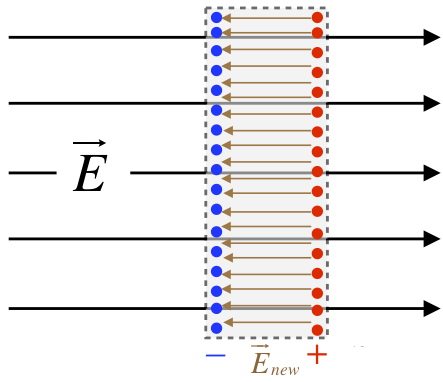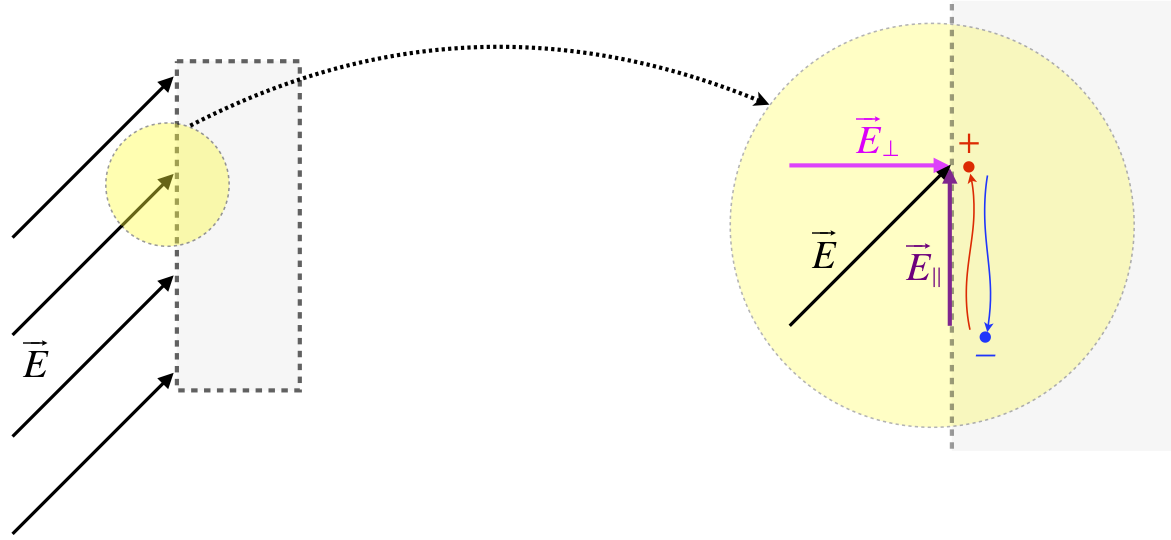# 1.5: Conductors

$$\newcommand{\vecs}{\overset { \rightharpoonup} {\mathbf{#1}} }$$ $$\newcommand{\vecd}{\overset{-\!-\!\rightharpoonup}{\vphantom{a}\smash {#1}}}$$$$\newcommand{\id}{\mathrm{id}}$$ $$\newcommand{\Span}{\mathrm{span}}$$ $$\newcommand{\kernel}{\mathrm{null}\,}$$ $$\newcommand{\range}{\mathrm{range}\,}$$ $$\newcommand{\RealPart}{\mathrm{Re}}$$ $$\newcommand{\ImaginaryPart}{\mathrm{Im}}$$ $$\newcommand{\Argument}{\mathrm{Arg}}$$ $$\newcommand{\norm}{\| #1 \|}$$ $$\newcommand{\inner}{\langle #1, #2 \rangle}$$ $$\newcommand{\Span}{\mathrm{span}}$$ $$\newcommand{\id}{\mathrm{id}}$$ $$\newcommand{\Span}{\mathrm{span}}$$ $$\newcommand{\kernel}{\mathrm{null}\,}$$ $$\newcommand{\range}{\mathrm{range}\,}$$ $$\newcommand{\RealPart}{\mathrm{Re}}$$ $$\newcommand{\ImaginaryPart}{\mathrm{Im}}$$ $$\newcommand{\Argument}{\mathrm{Arg}}$$ $$\newcommand{\norm}{\| #1 \|}$$ $$\newcommand{\inner}{\langle #1, #2 \rangle}$$ $$\newcommand{\Span}{\mathrm{span}}$$$$\newcommand{\AA}{\unicode[.8,0]{x212B}}$$

## A New Constant

The constant $$k$$ that we introduced in Coulomb's law, and is present in all of the solutions above, was convenient in the context for which it was introduced, but we will introduce a new constant here that we will use hereafter. There is no fundamental difference in using either of these constants – mostly the change is for cosmetic reasons. Without further ado, presenting the permittivity of free space:

$$\epsilon_o \equiv \dfrac{1}{4\pi k} = 8.85\times 10^{-12} \frac{C^2}{Nm^2}$$

Rewriting the magnitudes of the coulomb field and the fields of the infinite distributions in Section 1.3 in terms of this constant, we have:

$E_{point}\left(r\right) = \dfrac{q}{4\pi\epsilon_or^2}$

$E_{line}\left(r\right) = \dfrac{\lambda}{2\pi\epsilon_o r}$

$E_{plane} = \dfrac{\sigma}{2\epsilon_o}$

We will make extensive use of these formulas from this point on. We will also discard the use of $$k$$ in favor of $$\epsilon_o$$ in all forthcoming applications.

## Electrostatics

Before we discuss the effects of conductors on electric fields, it is essential that we make clear that we are proceeding under the following restriction: We are talking about electrostatics. This means that the charges present are in a state of static equilibrium – they are not moving, nor are they accelerating. We have already said that conductors allow for charges to flow freely, so how can these two things be reconciled?

If a conductor suddenly finds itself in the presence of an electric field, then the charges on that conductor will start to move as a result of the new force. As the charges move, the fields (which are affected by the placement of these charges) also change. The charges continue accelerating until the field contributions of the displaced charges cancel the external field. With zero net field, the charges no longer accelerate, and we will assume that their kinetic energy dissipates such that they also come to rest.

The conclusions we will draw here do not apply to the period of time when the charges are still moving around – we will only be considering the case when the charges finally reach an equilibrium electrostatic state.

## Inside Conductors

We characterized electric fields as "signals" sent out by electric charges. This is of course a model (as is all of physics), and this model does not make exceptions that allow for matter to "block" this signal. The only way to affect the field at a point in space caused by one charge is to introduce a field from another charge, such that the two fields superpose.

Let's now consider what happens when we suddenly introduce a uniform external electric field to a rectangular conducting slab. We represent uniform electric fields with parallel, equally-spaced field lines, and as we just said above, these field lines are not interrupted by matter, so the situation looks something like this:

Figure 1.5.1 – Conductor Introduced to FieldWhile the conductor is neutrally-charged, it is not without electrical charge – it is made of atoms, which are comprised of protons and electrons. This charge is (by definition of the conductor) free to move. This means that the positive charges in the conductor in the diagram (depicted as red) are pushed to the right by the electric field, while the negative charges (depicted as blue) are pulled to the left.

Figure 1.5.2 – Charges Migrate Because of Applied Field[Okay, so technically only the electrons – the negatively-charged particles – are free to move, or this conductor would not be a solid. This is a distinction that will not be at all important to us, because when negatively-charged electrons vacate a region, they leave an excess of positively-charged protons behind, which is completely equivalent to the positive charge moving into that electron-vacated region.]

The effect of this migration of charge is the creation of two planes of charge, one on each side of the slab (we will always assume that the field is not strong enough to pull the electrons off the surface of the metal). But this separation of charge itself has consequences with regard to the field. In particular, within the metal a new uniform field starts to develop. This field points away from the positive charges toward the negative charges, which opposes the direction of the external field.

Figure 1.5.3 – Internal Field Induced by Displaced ChargesWhen the field induced within the conductor is superposed with the applied field, the result is a weaker field within the conductor. The question is, how much weaker does it become? Suppose it only becomes a little weaker. That would mean that there is still some net field pointing left-to-right. But if this is the case, then more charge will migrate, making the induced field that points left even stronger, making the superposed field even weaker. In other words, we don't reach electrostatics until enough charge has moved to make the superposed internal field vanish. We therefore get the following remarkably general conclusion:

Electric fields vanish within conductors when the charges are static.

It's important to remember that this rule applies within the metal itself. If a conductor is hollow, the interior space does not qualify as being "within" the conductor, and the field is not necessarily identically zero.

## At the Surface of Conductors

The example above assumed that the uniform field made a right angle with the surface of the conductor, so let's generalize this a bit by allowing the field to make a different angle.

Figure 1.5.4 – Conductor Introduced to Field at an AngleWe handle this case as we would with any vectors – by breaking them into components. The component perpendicular to surface will affect the charges in the conductor exactly as described above – they will migrate to the two surfaces until the horizontal part of the field within the conductor vanishes. But what about the component parallel to the surface? This will only affect the charges at the surface, but again it will cause a migration. Here is a blown-up depiction of what is going on:

Figure 1.5.5 – Surface Migration of ChargesAs before, these displaced charges result in an induced field, which points from the positive charges toward the negative charges, opposing the parallel component of the external field. The charges keep migrating until the superposition of the induced field and the parallel component of the external field cancel each other. Unlike the case of inside the conductor, the whole field does not vanish in this case – just the parallel component does. The result is another remarkably general conclusion:

Electric field lines strike conducting surfaces at right angles.

The migrated charges create fields that extend out into the nearby space, and this causes the total electric field to adjust "smoothly" into the perpendicular surface strike:

Figure 1.5.6 – Field Lines Land OrthogonallyIt might appear from our specific example that this result only applies to flat conducting surfaces, but it is more general than that. The charges cannot remain static at any point on the surface of any conductor if the electric field at that point has a parallel component. So charges on a conductor rearrange themselves such that the total electric field lands at right angles to the conducting surface regardless of its shape.

## Field Strength at a Conducting Surface

Now that we know about the direction of an electric field at the surface of a conductor, let's use a simple model to have a look at the magnitude. We return to our example of a conducting slab with a perpendicular field (Figure 1.5.1), but here we will assume that the slab is infinite in extent (up/down and into/out of the page), with a finite thickness. When the charges migrate for this slab, they form two infinite planes of charge, for which we have computed the electric field strength. We found that these fields are uniform and are proportional to the charge density (Equation 1.3.22). Note that for every electron that migrates, it leaves behind a proton, which means that the charge density of both planes of charge are equal in magnitude (but have opposite signs). So our physical model has three separate electric fields that superpose: The applied electric field, the induced electric field of the negative plane of charge, and the induced electric field of the positive plane of charge. Putting these all together looks like this:

Figure 1.5.7 – Three Source Fields Near a Conducting SlabThe shaded area is the slab, and we are focused on the field to each side of the left surface. The external field has drawn the negative charges to this surface, while pushing positive charges to the other surface (or rather, leaving them behind). The magnitudes of the fields $$\overrightarrow E_–$$ and $$\overrightarrow E_+$$ are equal, and are, in terms of the charge density at each surface:

$\left|E_–\right| = \left|E_+\right| = \dfrac{ \left|\sigma\right|}{2 \epsilon_o}$

The fact that the field $$\overrightarrow E_–$$ points toward the negative charges and $$\overrightarrow E_+$$ points away from the positive charges means that the two fields point in the same direction inside the conductor, resulting in a total induced field inside the conductor with a magnitude of $$\dfrac{\sigma}{\epsilon_o}$$. But as we already know, the field inside the conductor vanishes, so we must have that enough charge must migrate such that:

$\left|E_{ext}\right| = \left|E_–\right| + \left|E_+\right| = \dfrac{\sigma}{\epsilon_o}$

Now let's look outside the left surface of the slab. In that region, the two induced fields are pointing in opposite directions. They are equal in magnitude, so they simply cancel each other, giving a total field strength equal to just the external field. Therefore we have for the total electric field outside the surface of the conductor:

$\left|E_{tot}\right| = \dfrac{\sigma}{\epsilon_o}$

While this appears to be a very specific result, we will shortly show that it applies to every conducting surface (not just an infinite slab). In such cases, the charge density can be different at different points on the surface of the conductor, but whatever the density happens to be at a specific point gives us the electric field at that point according to this equation. Given that we also know that the electric field is perpendicular to the surface at this point, we know everything there is to know about the field there. This is a strikingly simple and powerful result that we will use over and over.

This page titled 1.5: Conductors is shared under a CC BY-SA 4.0 license and was authored, remixed, and/or curated by Tom Weideman directly on the LibreTexts platform.